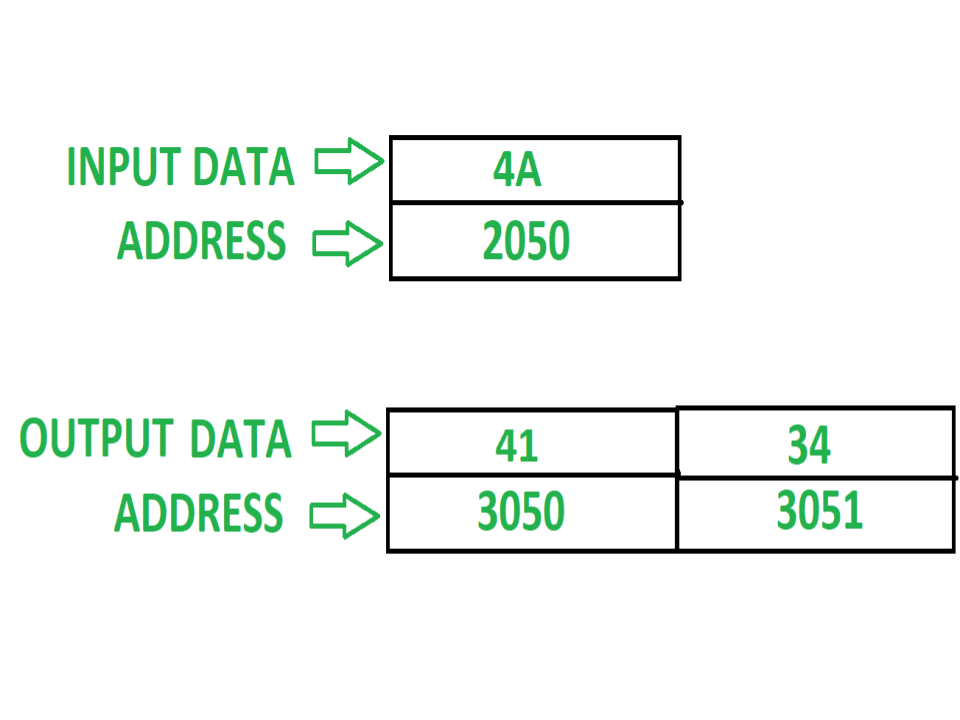# 8085 code to convert binary number to ASCII code

Problem – Assembly level program in 8085 which converts a binary number into ASCII number. Example –Assumptions – Binary number which have to convert in ASCII value is stored at memory location 2050 and output will be displayed at memory location 3050 and 3051. Algorithm –

1. Load the content of 2050.
2. Then separate the LSB of the no. using ANI 0F instruction and MSB of the number by again loading the content of 2050 and rotate it by one bit 4 times to get reverse of the number and then again use ANI 0F to separate the digit.
3. If the digit is more than or equal to 0A (in hexadecimal) then add 37 otherwise add 30 to convert into ASCII value (For checking the number is greater than or equal to A then use instruction: CPI 0A and then check the carry flag, if it is 0 then it means digit is greater than or equal to A and if 1 digit is less than A).
4. Now Store the ASCII values of both the digits in 3050 and 3051 respectively.

Program – Main routine:

Sub routine:

Explanation – Main routine:

1. LDA 2050: This instruction will load the number from address 2050 to the accumulator.
2. CALL 2500: This instruction will stop executing the main routine instructions after it and will move to the subroutine address 2500 for performing the subtask and after performing subroutine instructions it will come back to mainroutine and execute the instructions after CALL 2500.
3. STA 3050: This instruction will store the result (performed in subroutine) of Accumulator to address 3050.
4. LDA 2050: This instruction will again load the number from address 2050 to the accumulator as the earlier loaded number is changed in accumulator.
5. RLC: Rotate the contents of Accumulator by one bit left side without carry.
6. RLC: Rotate the contents of Accumulator by one bit left side without carry.
7. RLC: Rotate the contents of Accumulator by one bit left side without carry.
8. RLC: Rotate the contents of Accumulator by one bit left side without carry. (Applying RLC 4 times it will reverse the contents of the Accumulator)

9. 9. CALL 2500: This instruction will stop executing the main routine instructions after it and will move to the subroutine address 2500 for performing the subtask and after performing subroutine instructions it will come back to mainroutine and execute the instructions after CALL 2500.
10. 10. STA 3051: This instruction will store the result (performed in subroutine) of Accumulator to address 3051.
11. 11. HLT: This instruction will terminate the program.

Sub routine:

1. ANI 0F: This instruction will separate the LSB of the number present in Accumulator and stores the result back in Accumulator.
2. CPI 0A: This instruction will compare the content of Accumulator with 0A i.e. [A]-0A.
3. JNC 205A: If the carryflag becomes 0 then it will jump to 205A otherwise move to the next instruction.
4. ADI 30: It will add 30 to the content of Accumulator and again store the result back in Accumulator.
5. RET: Now it will move back to the main routine after the next instruction of CALL and start executing instructions of main routine.
6. It will add 37 to the content of Accumulator and again store the result back in Accumulator.
7. RET: Now it will move back to the main routine after the next instruction of CALL and start executing instructions of main routine.

### Advantages of converting binary number to ASCII code:

1. Converting a binary number to ASCII code is a common operation used in many applications, such as displaying binary data on a computer screen or printing binary data to a printer.

2. It is a simple and straightforward task that can be easily implemented in any programming language.

3. It is a fast operation that can be completed in O(n) time complexity, where n is the number of bits in the binary number.

### Disadvantages of converting binary number to ASCII code:

1. If the binary number is very large, storing the entire number in memory may not be feasible, which could require a more complex solution such as processing the binary number in smaller parts.

2. If the binary number is negative, additional logic is required to handle the sign bit and convert it to a negative ASCII code.

3. If the ASCII code is being displayed on a computer screen or printed to a printer, the font used to display the characters may not be able to display all ASCII codes correctly, which could result in distorted or unreadable characters.
Whether you're preparing for your first job interview or aiming to upskill in this ever-evolving tech landscape, GeeksforGeeks Courses are your key to success. We provide top-quality content at affordable prices, all geared towards accelerating your growth in a time-bound manner. Join the millions we've already empowered, and we're here to do the same for you. Don't miss out - check it out now!

Previous
Next